Next: Solving univariate polynomial numerically Up: Implementation Previous: Implementation   Contents

Example

The program Test_Solve_UP is a general test program which enable to solve univariate polynomial whose coefficients are given in a file by increasing power of the unknown.

We use as example the Wilkinson polynomial of degreewhere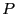: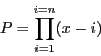It is well known that this polynomial is extremely ill-conditioned. For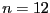the coefficient of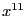is 78. But if we modify this coefficient by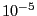there is a big change in the roots, 4 of them becoming complex . The general procedure leads to reasonable accurate result up to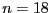. At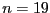although Kantorovitch theorem has determined interval solutions that indeed contain all the solutions, Newton method is unable to provide an accurate estimate of this root due to numerical errors.

For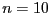and if we are looking for the roots in the interval [0,2] the computation time is 90ms, for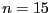190ms and 330ms for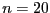. For the fast algorithm these times are: 10ms, 20ms, 30 ms Note that the best classical solving algorithm start to give inaccurate results for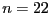(between 12.5 and 18.5 the interval analysis algorithm finds the roots 13.424830, 13.538691, 15.477653, 15.498664, 17.554518, 17.553513) and give imaginary roots for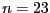.Next: Solving univariate polynomial numerically Up: Implementation Previous: Implementation   Contents
Jean-Pierre Merlet 2012-12-20